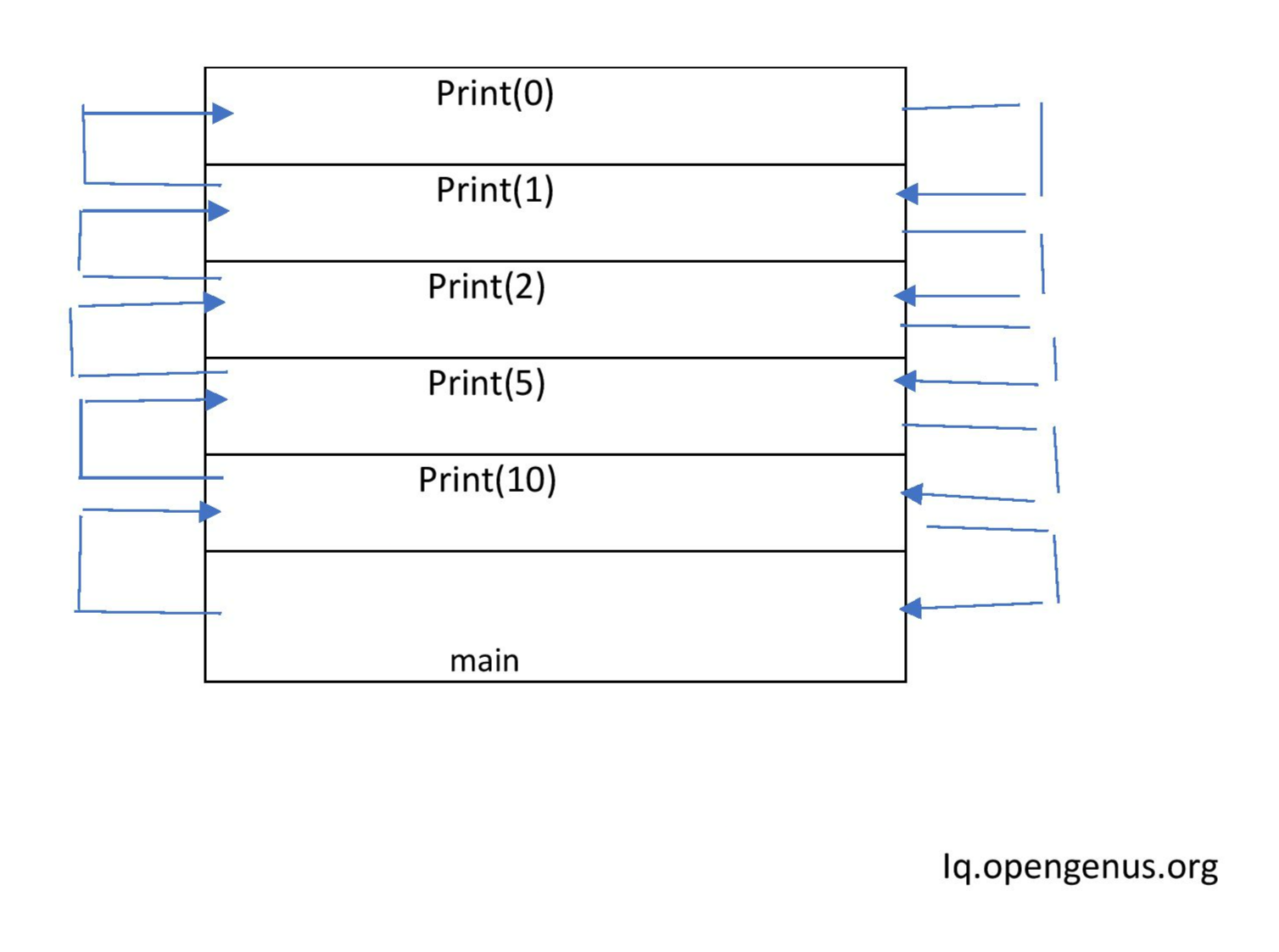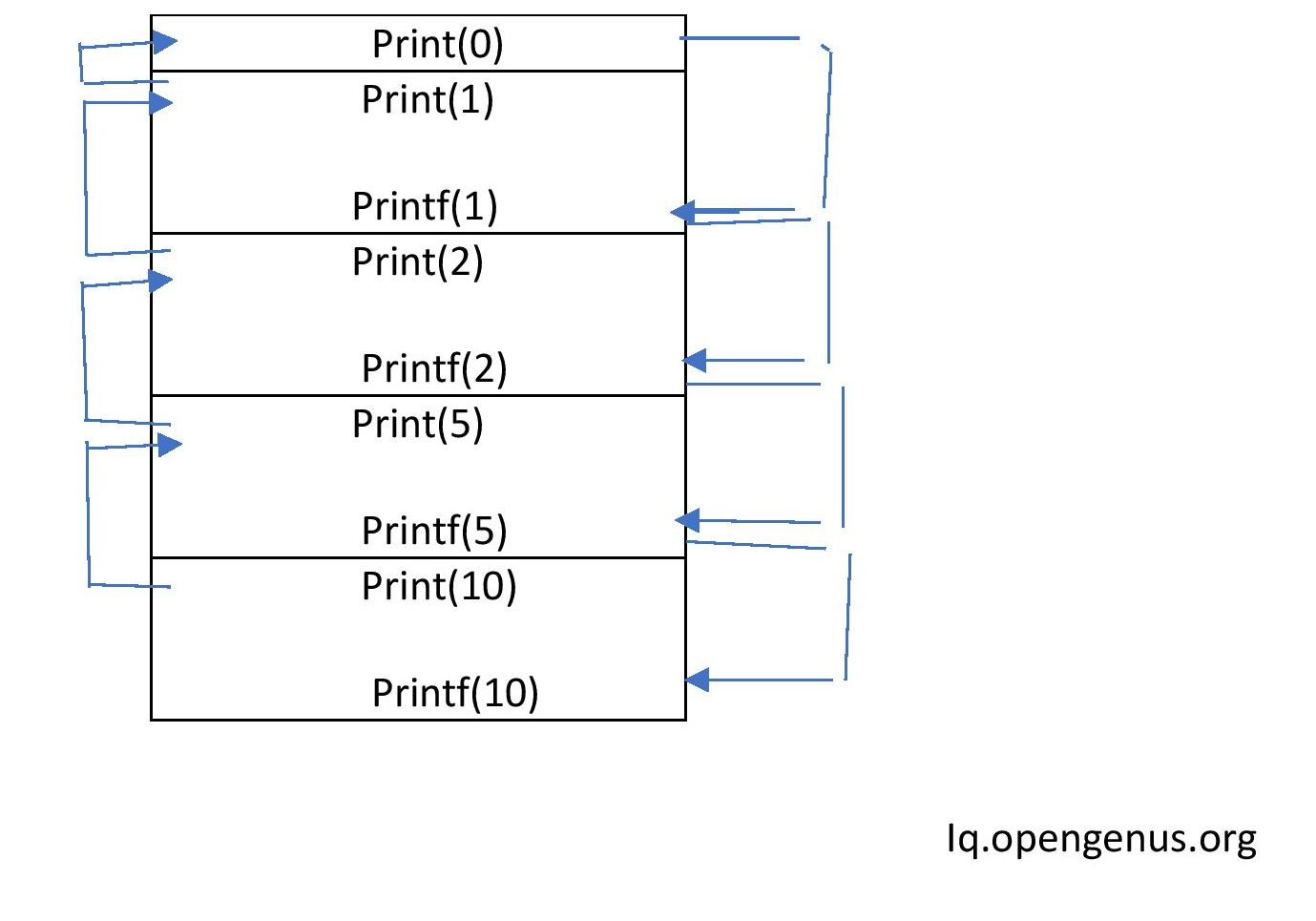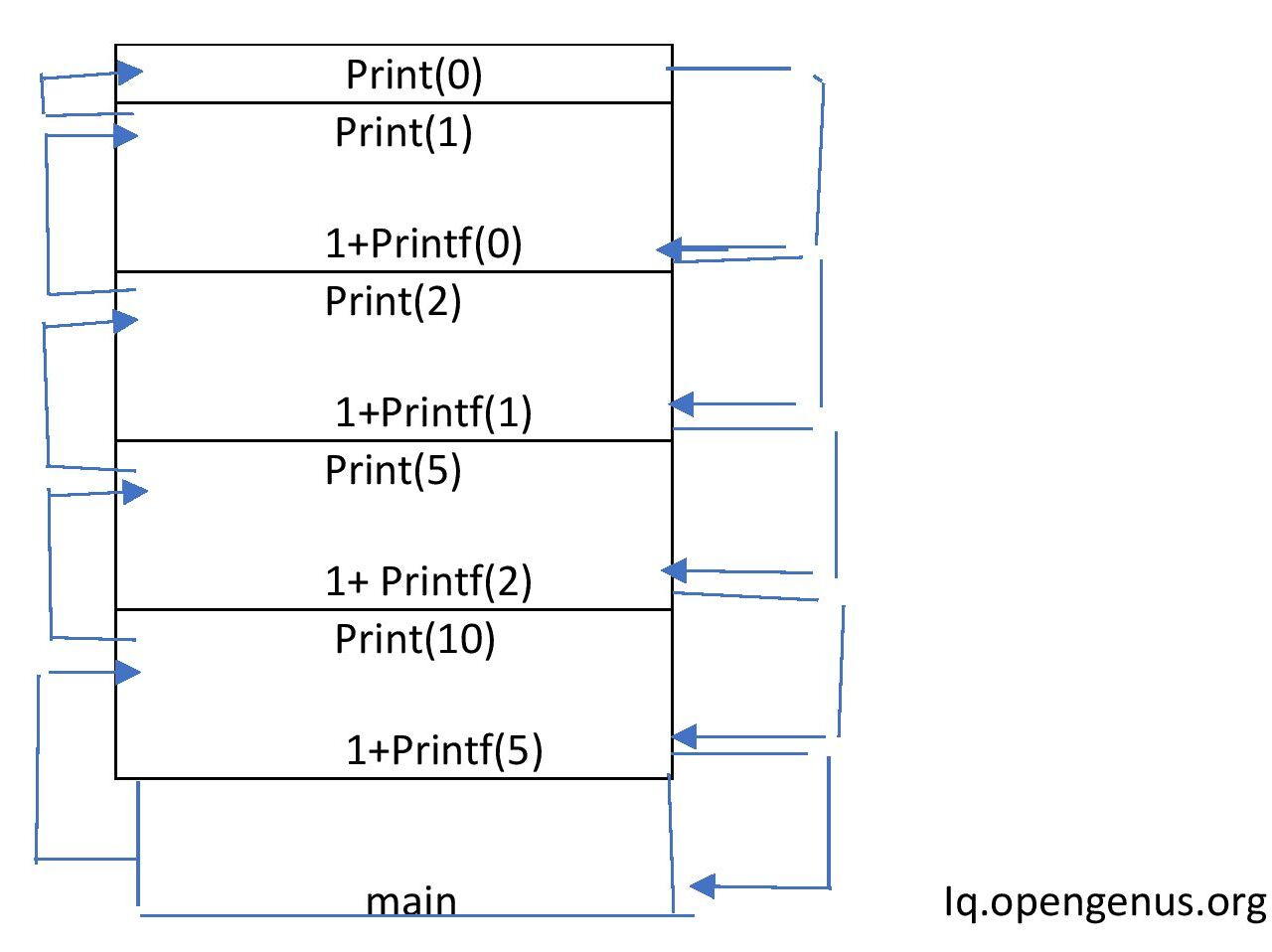×

Search anything:

# Tail Recursion

#### Algorithms recursionFREE BOOK -> Problems for the day before your Coding Interview (on Amazon)

In this article, we have explored the idea of Tail Recursion in depth with several code examples along with comparison with Non-Tail Recursion.

# Contents

1. Introduction
2. Properties
3. Tail and Non-Tail Recursion
4. Examples Of Tail Recursion.
5. Logic for finding Tail or Non-Tail Recursion.
6. Examples Of Non Tail Recursion.
7. Function that look like Tail recursion but is not.
8. Tail VS Non-Tail Recursion

# Introduction

What does the word tail mean? It marks the end.For an aircraft it is the tail of the vehicle which is the end part of the vehicle,that is the last thing. So, Tail recursion means recursion is last thing in the function.

# Properties

Recursion will be the final thing that is,there will be no task after the recursive call.The definition can be easily derived from the word Tail recursion itself.Recursion that acts as a tail -- end of a function.No task left after execution of recursive call.

# Tail and Non-Tail Recursion

In tail recursion the recursive call will be the last task but in non tail recursion there will be still some task left after the recursive function call,that is it is not the tail or end.

# Examples Of Tail Recursion.

``````#include <stdio.h>

void print(int a)
{
if(a<1)
{
return;
}
else{
printf("%d",a);
printf(" ");
print(a/2);
}
}

void main()
{
print(10);

}
``````

In print(int a) function ,print(a/2) is the last statement which is a recursive call.So by looking at this we can tell that it is tail recursion as it is the last thing here in the function.

It is true here.But it is not always like that,in some function it looks
like the recursive call is the last thing written.It looks like tail recursion but still it is non-tail recursion.

So,we will discuss the correct logic to find tail or non-tailrecursion.

# Logic for finding Tail or Non-Tail Recursion

For this let us again take the same code we gave as an example for tail recursion:

``````#include <stdio.h>

void print(int a)
{
if(a<1)
{
return;
}
else{
printf("%d",a);
printf(" ");
print(a/2);
}
}

void main()
{
print(10);

}
``````

Let us see the execution of this code:

First the control will go to main function where we are calling the function print(10).

As recursion is happening the use of stack is involved.First activation record for main.In main we will call print(10).The control will go to the print function and one more activation record for print(10) will be push to the stack.

As a=10 not less than 1 it will go to else part and will print 10 and again it will call print() by passing a/2=10/2=5 that is print(5).Therefore,one more activation record for print(5) is pushed to the stack.

As 5 is not less than 1 it will print 5 and will again call print function as print(5/2)=print(2).

Again an activation record for print(2) is pushed to the stack.
As 2 is not less than 1 it will print 2 and will again call print function as print(2/2)=print(1).

An activation record for print(1) is pushed to the stack.
As 1 is not less than 1 it will print 1 and will again call print function as print(1/2)=print(0).

Again an activation record for print(0) is pushed to the stack.
Here 0 is less than 1 ,so it will return and not gonna execute the else part.We are going to return to where print(0) was called,that is to print(1) record.No other statement to execute after the recursive callSo,it will returnback to print(2) from where it was called.Similarly,it will return to print(5),then to print(10),then to the main function.The memory will be freed when we return back.

It will print the result as 10 5 2 1.## Output

``````10 5 2 1

...Program finished with exit code 0
Press ENTER to exit console.
``````

After the recursive calls ,no task is left to be done.So this is tail recursion.

# Examples Of Non Tail Recursion.

``````#include <stdio.h>

void print(int a)
{
if(a<1)
{
return;
}
else{
printf("%d",a);
printf(" ");
print(a/2);
printf(" ");
printf("%d",a);
}
}

void main()
{
print(10);

}
``````

In print() function print(a/2) is not the last task we have printf statement after that.So it is non tail recursion.

So,the stack will look like:## Output

`````` 10 5 2 1  1 2 5 10

...Program finished with exit code 0
Press ENTER to exit console.
``````

# Function that look like Tail recursion but is not.

``````
#include <stdio.h>

void print(int a)
{
if(a<1)
{
return;
}
else{
return 1+print(a/2);
}
}

void main()
{
int x;
x=print(10);
printf("%d",x)
}

``````

return 1+print(a/2) is the last statement .It appears as Tailed recursion but is actually non tail.After the function call we have task to execute that is add 1 to the return of recursive call and then return .So adding of 1 is the last step not the recursive call.So it is non tailed recursion.

# Tail VS Non-Tail Recursion

We usually maintain a stack if after returning there is something to be executed but in tail recursion,if we look at the stack there is nothing to be executed after returning from recursive call.Then why are we maintaining stack.it is waste of our precious memory.

So,tail programs are ver much like loops.It is better to write the same program using loops.Time complexity will be same.But space complexity using tail recursion is O(n) and using loop is O(1).

Some compilers automatically optimise the code.When they see that the recursion is tail recursion they will not maintain stack as no need to keep addresses of the function calls.

So,tail recursion is not a good option to write programs because of the memory space it takes.

But in non tailed recursion by observing the execution flow we can see that we are fully utilising the stack.We have to maintain the stack as after returning also we have to do something (here add 1).

With this article at OpenGenus, you must have the complete idea of Tail Recursion.